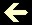CIRCULATING DECIMAL FRACTIONS

Question: Which is the vulgar fraction that generates the circulating decimal 12.345 6789 6789 6789...?

Answer: 123 444 444 / 9 999 000

I reveal the secret formula to decipher the vulgar fraction for any circulating decimal fraction:Use the script below, if you'll prefer:

Input:
Int - the integer part of the number, if it exists
Frac - the non-repeating fractional part, if it exists
Rep - the repeating fractional part (circulating decimal) just once:

 Int , Frac Rep Dividend Divider
 Dividend/Divider